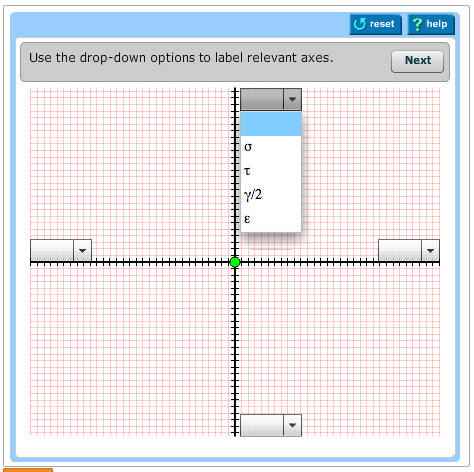### Create an Account

Already have account?

### Forgot Your Password ?

Home / Questions / Part A Construction of MohrA1s circle for the state of stress for the yz plane For the e...

# Part A Construction of MohrA1s circle for the state of stress for the yz plane For the element shown construct MohrA1s circle for the state of stress for the yz plane Use the graph

Part A - Construction of MohrA????1s circle for the state of stress for the y-z plane

For the element shown, construct MohrA????1s circle for the state of stress for the y-z plane. Use the graph provided.

First, select the center of MohrA????1s circle (point C) and select the point that represents the state of plane stress shown (point A). Given these two points, determine the length of the line between these two points, which represents the radius R of the MohrA????1s circle.Part C - Three-dimensional state of stress for the element

Using the MohrA????1s circle constructed, determine the three-dimensional state of stress (?max, ?int, ?min, and ?absmax) for the element.

Express your answers in psi to four significant figures separated by commas.

Show transcribed image text Part A - Construction of Mohr's circle for the state of stress for the y-z plane For the element shown, construct Mohr's circle for the state of stress for the y-z plane. Use the graph provided. First, select the center of Mohr's circle (point C) and select the point that represents the state of plane stress shown (point A). Given these two points, determine the length of the line between these two points, which represents the radius R of the Mohr's circle. Part C - Three-dimensional state of stress for the element Using the Mohr's circle constructed, determine the three-dimensional state of stress (?max, ?int, ?min, and ?absmax) for the element. Express your answers in psi to four significant figures separated by commas.

Jun 24 2020 View more View Less

#### Answer (Solved)Subscribe To Get Solution